﻿ Chapter 9: Analysing relationships between variables using STATA

# Chapter 9: Analysing relationships between variables using STATA

### Box 9.3 Presenting the results for Pearson’s correlation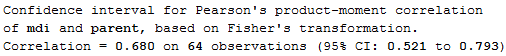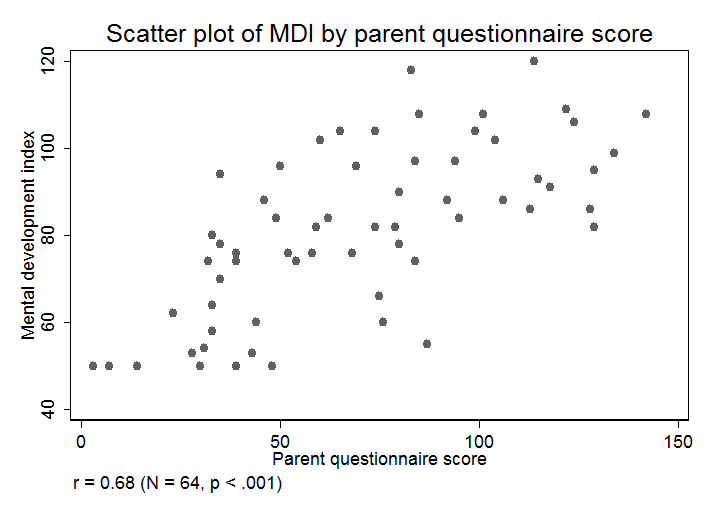### Figure 9.1, Figure 9.2 and Box 9.3 Code### Figure 9.3 Scatterplots for several variables### Figure 9.3 Code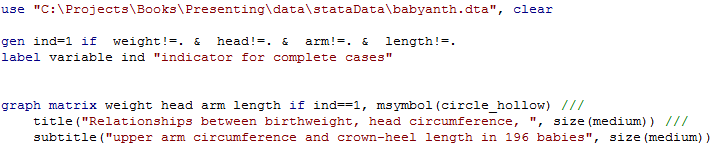### Figure 9.4 Calculating several correlations### Figure 9.4 Code### Figure 9.5 Presenting scatterplots (a) with skewed data and (b) where data are transformed### Figure 9.5 Code### Figure 9.6 Output for a rank test### Figure 9.6 Code### Figure 9.7 Scatterplot of two variables with linear regression line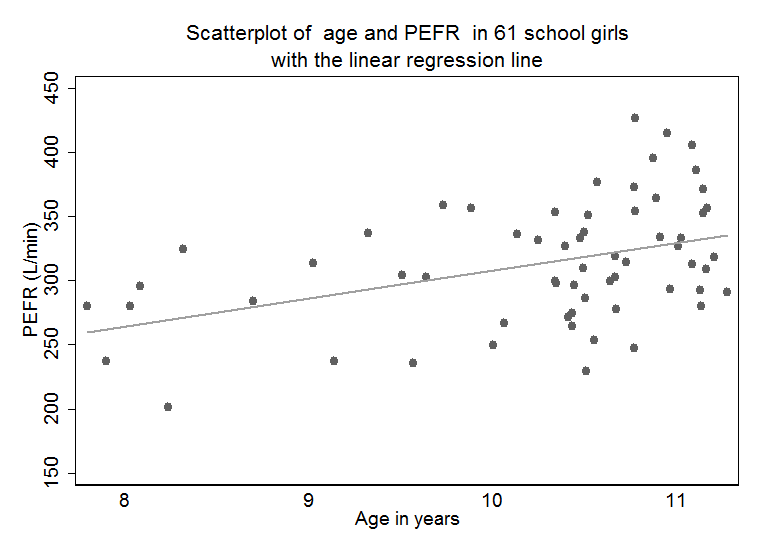### Figure 9.7 Code### Figure 9.8 Output for simple regression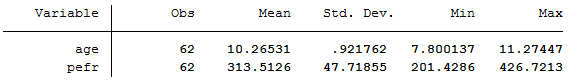### Figure 9.8### Box 9.11 Presenting the results for several predictor variables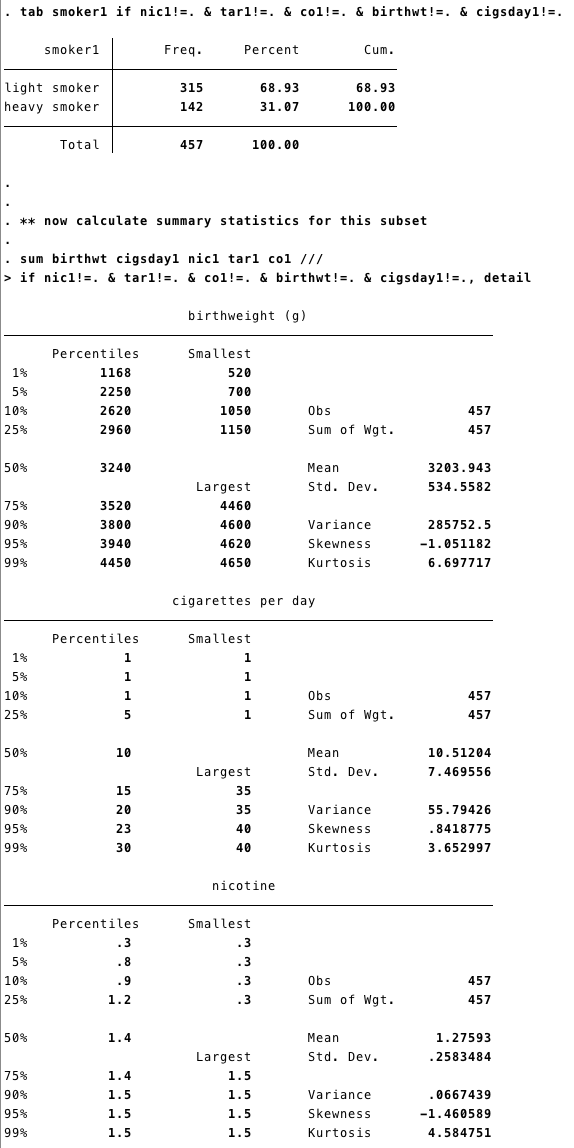### Box 9.11 Code